# Problem of the Day March 30, 2020

Mar 30, 2020March 30, 2020 Problem of the Day

Grade 6: Convert the following %s into decimals and fractions in simplest form:   20%,  35%,  72%,  175%,  5%

Grade 7: Solve the following problems: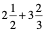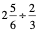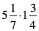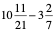Grade 8: Find the mean, median, mode and range of the following set of numbers: 2, 7, 11, 11, 16, 24, 29, 33

Algebra: Solve the following problems: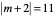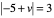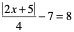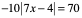Geometry: Find the midpoint and the distance between for the following points:   (5 , 7) and (-3 , 5)       (9 , 4) and (3 , 5)

Algebra 2: Solve the following inequalities:

-4 < m - 5 < 2               9 + 2b < 7  or 7 - 5b < -8

Trigonometry: Solve the following equations: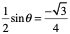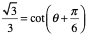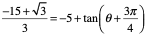Pre-Calculus: Use the properties of logarithms to expand the following logarithmic expressions: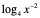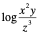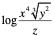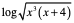Grade 6: 0.2,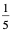0.35,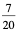0.72,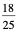1.75,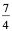0.05,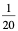Grade 7: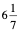,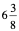,         9,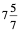Grade 8: mean 16.625  median  13.5  mode 11  range 31

Algebra:  9, -13               8, 2                27.5,  -32.5           no solution

Geometry: midpoint (1,6) distance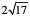midpoint (6, 4.5) distance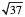Algebra 2:    1<m<7          b<-1 or  b>3

Trigonometry: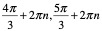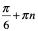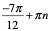Pre-Calculus: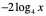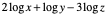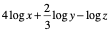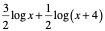,